# Electric Fields

## Coulomb’s Law

Electric Force

Coulomb’s law states that the electric force is directly proportional to the product of the charges and inversely proportional to the squared distance between them.__F __= Force (N)

ε0= Permittivity of free space

__Q __= Charge of one object (C)

q = Charge of second object (C)

__r __= Distance between them (m)

The permittivity of free space is a measure of how well a field permeates, or spreads throughout, a region. In this case we are going to treat the permittivity of air as being equal as that of a vacuum (free space).

## Electric Field Strength

Drawing Electric Fields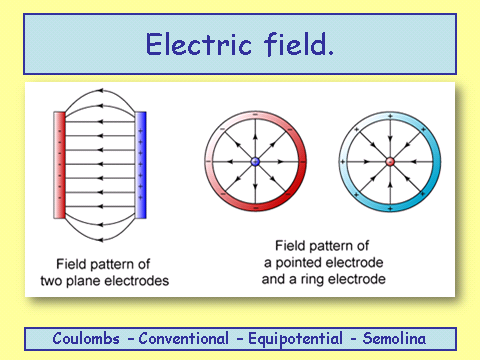Notice the direction of the field lines. They are pointing from positive and going to the negative plate.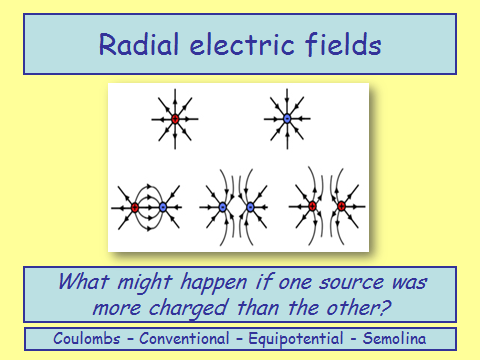If one source was more charged and therefore had a larger electric field, the points at which the resultant force is zero would be shifted towards the weaker charge.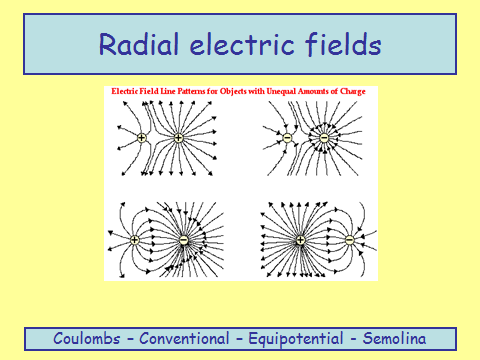Electric Field Strength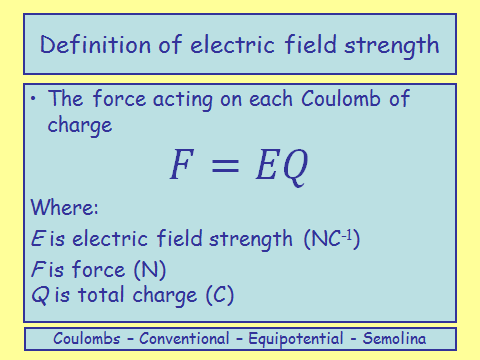Work Done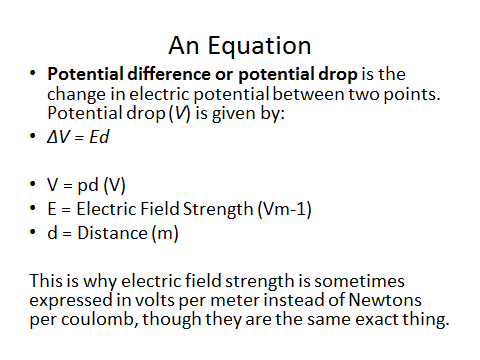Moving Charges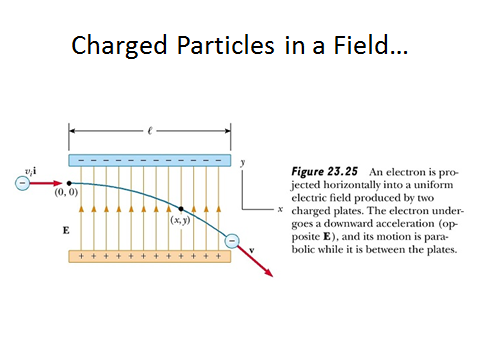Notice that the shape of the trajectory is parabolic.

Electric Field Strength 2

The magnitude of the electric field strength is similar to that of the gravitational field in design;## Electric Potential

Electric potential works the same as gravitational potential. It is the work done when moving an object from infinity to a point in the field.

Similarly electric potential difference is the work done in moving a charge from one point to another point in an electric field.

Work Done

Yes - this is similar to gravitational work done.

Equipotential Surfaces

The dotted green lines are the lines of equipotential.

Electric Potential and Electric Field Strength

Electric potential and electric field strength are related by the following relation: